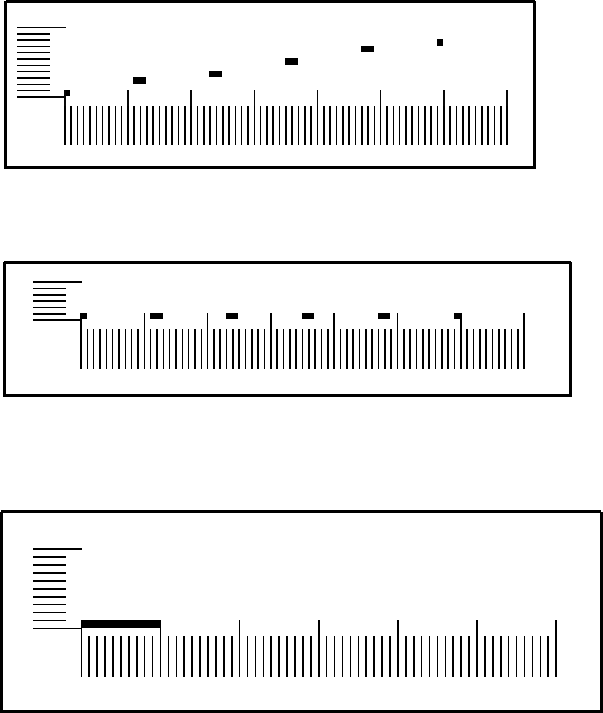Next: Four-dimensional Hypersurface Up: USING N-LAND: CASE STUDIES Previous: USING N-LAND: CASE STUDIES

Three-dimensional Line

The first data set examined is a sampling of points from a line in three-dimensional space. While it would be much faster in this case to simply draw the points using standard 3-D graphics techniques, the example is useful to help appreciate the search strategies used in N-Land. Figure 3a shows an integer sampling of a line in three-dimensional space going from point (0, 0, 0) to (9, 9, 5). The tick marks indicate discretization of each dimension, with the longer marks indicating changes in the slower dimensions. Intuitively, there should be a way to look at this line that shows all the points aligned together.

Bringing the line into parallel with one of the major viewing planes should cause the points to line up, so the first shear chosen was a negative 60 degree shear, with dimension 3 (Z axis) as the shearing axis and dimension 2 (Y axis) as the fixed axis. The results are shown in Figure 3b.

Finally, a shear of negative 30 degrees is applied, this time using dimension 3 as the fixed axis and dimension 1 as the shearing axis. This brings the points into alignment, and after clipping creates a coherent line, as seen in Figure 3c.Next: Four-dimensional Hypersurface Up: USING N-LAND: CASE STUDIES Previous: USING N-LAND: CASE STUDIES
Matthew Ward
1999-02-23